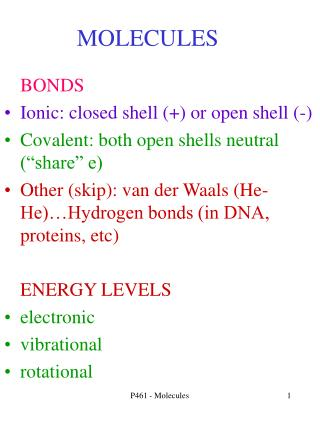DownloadDownload PresentationMOLECULES

# MOLECULES

Download Presentation## MOLECULES

- - - - - - - - - - - - - - - - - - - - - - - - - - - E N D - - - - - - - - - - - - - - - - - - - - - - - - - - -
##### Presentation Transcript

1. MOLECULES • BONDS • Ionic: closed shell (+) or open shell (-) • Covalent: both open shells neutral (“share” e) • Other (skip): van der Waals (He-He)…Hydrogen bonds (in DNA, proteins, etc) • ENERGY LEVELS • electronic • vibrational • rotational P461 - Molecules

2. Ionic Bonds - NaCl • First approximation. Both atoms are ions (no electron sharing) and bond due to EM force between two charged bodies • Atom valence ionization ~effZ radius • Na 3s 5.1 eV 1.8 .17 nm • Cl 3s23p5 13 eV 2.9 .07 nm • Ar 3s23p6 16 eV 3.3 .07 nm • Ar more tightly bound than Cl. But Cl- “looks” like Ar. Has effective Z ~ 3 P461 - Molecules

3. Ionic Bonds - NaCl • There is an EM attraction between the two ions • if |U| > (5.1-3.8) eV = 1.3 eV, then can have a bound NaCl molecule • but the two ions can’t get too close. Electron’s wave functions begin to overlap. Some states will become filled and Pauli exclusion forces to higher energy (large gap) • size of filled 2p about 0.05 nm • the nuclei will start to not be shielded from each other --> some ++ repulsion P461 - Molecules

4. Ionic vs Covalent • As R >> 0.05 (size of 2p orbit), there is little overlap in the electron wave function between the Na and Cl ions ---> mostly ionic bond “94% ionic and 6% covalent (DH makes up numbers) • look at HFl molecule H ionization energy = 13.6 eV Fl electron affinity = 3.4 eV -----> need 10.2 eV in electrostatic energy • as the size of filled 2p in Fl about 0.05 nm and the nominal 1s in an H atom is 0.05 nm, the electrons are attached to both atoms --> covalent bond “10% ionic and 90% covalent” (DH made up numbers) • the nuclei will start to not be shielded from each other --> some ++ repulsion P461 - Molecules

5. Covalent Bonds - Diatomic Molecules • assume all valence electrons are shared • often S=0 lowest energy but not always (Oxygen is S=1) • if both atoms are the same then |y|2 same if switch atom(1) and atom(2) --- electron densities around each atom are the same (even sort of holds if different atoms like CO) • H(1s) <-- very far apart ---> H(1s) • close together H(“1s”)H(“1s”) • electron wavefunctions overlap -“shared” • two energy levels (S=0,1) which have E bands R=infinity (atoms) 1s*1s Vib and rot P461 - Molecules

6. Covalent Bonds - Hydrogen • even if only 1 electron, bond is covalent • look first at ionized diatomic H • have repulsive potential between 2 protons depends on R = p-p separation (about 0.11 nm) • guess at a 3D solution for the wave function • at large separation just two H atoms • two possibilities: symmetric and antisymmetric when the separation becomes small P461 - Molecules

7. Covalent Bonds - Hydrogen+ • symmetric wave function has lower energy • less curvature. 1 “node” vs 2 “nodes” (compare to particle in a box) • also greater shielding of protons from each other as higher probability for the electron to be between the two protons (antisymmetric goes to 0 at midpoint) • can extrapolate to R=0 --- symmetric becomes a 1S state of He and antisymmetric (with wavefunction=0 at orgin) becomes a 2P state • determine this as a function of R internuclear separation. Find there is a minimum for symmetric but not for antisymmetric---> “covalent” bond P461 - Molecules

8. Energy Levels • for given electronic states (1s,3p, etc S=0, S=1) determine effective V(R) and see if a minium (bound state) exists • as NOT V(r) potential, Sch. Eq. Not separable into (THETA,PHI) parts • ---> L2 not eigenfunction, L not good eigenvalue • but often phi symmetry --> Lz m “good” • will then have H.O. vibrations around minimum R=nuclear separation V P461 - Molecules

9. Neutral Hydrogen Molecule • more tightly bound with 2 electrons. Have: • additional shielding of protons (lower E) • e-e repulsion (higher E) • end up: R=0.07 nm (compared to about 0.09 nm with single electron) • the “size” of a H atom is about 0.05 nm and so the 1s wavefunctions of the 2 atoms are overlapping and need to use Fermi-Dirac statistics --> Pauli exclusion and a totally antisymmetric wavefunction P461 - Molecules

10. Neutral Hydrogen Molecule • the antisymmetric space has y=0 when r1=r2 • gives: lower electron probability between protons • less shielding --> higher energy • in this case (and in most cases) have covalent bond when electrons are paired with “antiparallel” spin S=0 S=1 S=0 P461 - Molecules